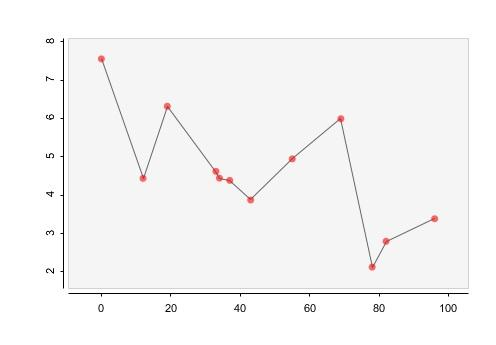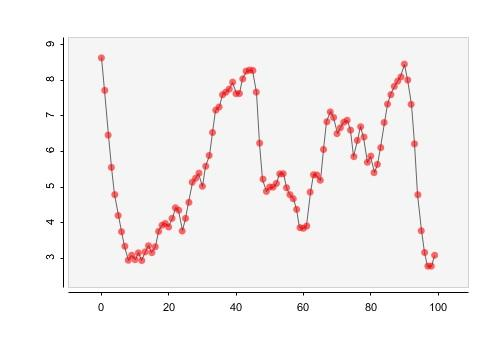#### Howdy, Stranger!

We are about to switch to a new forum software. Until then we have removed the registration on this forum.

# Interpolating points between dates on a graph

edited March 2017

So i'm trying to create a graph with the grafica library, and I have preset data with x/y axis already. The x axis is a timeline of dates at random intervals. What's the best to interpolate the points so that every day has an interpolated point so the graph appears smooth.

To see an example of how my graph currently looks, check out this example:

``````import grafica.*;

GPlot plot;
GPointsArray myArray = new GPointsArray(0);
int nPoints = 100;

void setup() {
size(500, 350);

plot = new GPlot(this, 0, 0, 500, 350);
myArray = new GPointsArray(nPoints);

for (int i = 0; i < nPoints; i = i + (int)(random(1,15))) {
}

plot.setPoints(myArray);
plot.defaultDraw();
}
``````

It will come out something like this:It should come out like this where instead of points every 1-15 ticks, it would be every tick.Edit: And I read about lerp() and map() but i'm not sure how to actually apply it

Tagged: